The 15-Puzzle (program description)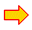to help pagedownload 15-puzzle programsee Delphi(7) project

Introduction

The 15-puzzel was around 1870 invented in New England and became very popular after 1880,
sweeping across America and Europe.
Below are two pictures of 15-puzzles, the left in an initial-, the right in the final (solved) state.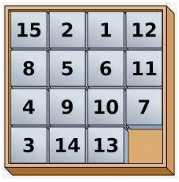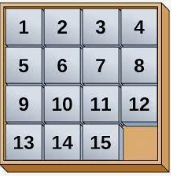A 4x4 square holds 15 tiles numbered 1 to 15 and one empty space.
A tile may not be lifted but can shift to its neighbouring empty space.
A puzzle is solved by shifting tiles until the solved state is reached in which the tiles are sequentially ordered.
This Delphi project allows for generating 15-puzzle games with selectable difficulty,
solving a game manually or have the computer search for solutions.
It helps the user to develop strategies.

Games together with solutions may be saved to disc.

For the computer search choice is between breadth-first or depth-first search.
15-puzzles are not difficult to solve, however finding the shortest solution is very hard.
It has been verified that 4*4 size puzzles can be solved in 80 moves or less.
Despite the simplicity of this puzzle, much computer time is needed.
Searching for a solution takes about t = 0.136*2.1^s microseconds
where s is the search depth in moves. (3GHz clock).
To explore 20 moves deep requires about 400 milliseconds,
this time increases to 10 minutes for a 30 moves deep search and about doubles for each extra move.

This illustrates the impossibility to find the shortest solution for hard puzzles by brute force:
80 moves would take a single processor longer then the time that our solar system exists.
So, in this project we are limited to approximations of the shortest solution.

Not every puzzle is solvable.

It is rather simple to shift the -1-tile to the left top corner, the -2- tile right next to it, etc.
Finally then we end up with 3 remaining tiles and 1 empty space in the right bottom corner.
These 3 tiles (11,12,15, empty) have 4! = 24 sequences but only the 12 below lead to a solution:A single exchange of two tiles in above picture makes the puzzle unsolvable.
To distinguish solvable and unsolvable puzzles needs a variable that is invariant to moves.
Calculating this variable for the solved game then sets the solvability criterion.
Here is the algorithm:
1. Write the tiles left-top to right-bottom as a permutation (sequence)
2. Count the number of inversions (an inversion is a pair a,b where a > b)
3. Add �1� if the empty space is at an even row (counting rows 1,2,3,4)
A solvable puzzle has odd parity sum:
1,2,3,4,5,6,7,8,9,10,11,12,13,14,15 ����0 inversions, +1 for empty space at row 4
Moving the empty space horizontally does not change anything.
Moving the empty space upward :
1,2,3,4,5,6,7,8,9,10,11,13,14,15,12 ���..1 inversion (12,15) empty space at row 3
Odd parity, solvable puzzle.

The following game has no solution: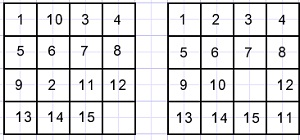Left is shown the original state, right the final (unsolvable) state.
The permutation is 1,10,3,4,5,6,7,8,9,2,11,12,13,14,15
The right picture shows the game where all tiles except 11,12,15 are placed in position.
The number of inversions is:
For the 10 at position 2: count = 8 (10 compared to ,3 4 5 6 7 8 9 2)
For the 2 at position 10: count =7 (2 compared to 3 4 5 6 7 8 9)
1 more for the empty space at row 4.
Together 8+7+1 = 16, unsolvable puzzle.

Coding the game

The tile and empty space positions on the board are saved as a one dimensional array[0..16].
[1..16] hold the tile number for that position.
 holds the position of the empty space.
A complete game is coded as an array of board positions.
Board positions:(right bottom is position 16)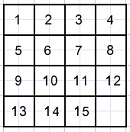```const maxmove = 250;
var game : array[0..maxmove,0..16] of byte;
moveNr : byte;//the number of moves, points to game[moveNr,1..16]
moveCount : byte;//total moves done
```
Game[0,0..16] holds the original puzzle, Game[1,0..16] is the board state after one move, etc.
So, game[moveNr,0..16] is the current board state.
This coding is convenient for step by step showing of a solution.

Solving a game

The above coding is inconvenient for puzzle solving.
Here a counter is needed for a systematic search.
A way is to move the empty space and save the direction of moves as digits of a counter.
Move directions: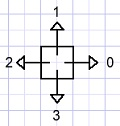Array gamedirections[1..16] holds a byte for each position.
A byte has a bit set if the move direction for that position is allowed.
```const gamedirections : array[1..16] of byte =
(\$9,\$d,\$d,\$c,\$b,\$f,\$f,\$e,\$b,\$f,\$f,\$e,\$3,\$7,\$7,\$6);
//bit 0..3 set if direction allowed for this position
var Xfield : byte; //0 element position
Xmove : array[0..maxmove] of byte;//directions list
XNr : byte;  //current trial move number
Xdir : byte; //trial move direction
PXnr : byte; //needed for breadth first search, see later
```
Before searching for a solution, array XGame[1..16] is copied from the Game[n,1..16] array.
Xfield = Game[n,0] holds the position of the empty field.
The Xmove[ ] array holds the subsequent moves (directions) of the empty field.
For the left top corner this value is \$9 = 1001 binary, directions 0 and 3 are allowed.
So, the search for a solution is disjunct from the game[ , ] array.
In case of a failing search for a solution the search maybe reselected
but now with another search depth or tile selection.

Partial solutions

To find a solution requiring 30 moves takes about 10 minutes.
For each extra move this time doubles.
How to solve more difficult games?
The answer is to search first for game states that have only a few tiles at the right place,
starting normally with tiles 1,2 .
The next search than tries to position the 3,4 tiles at the right place,
then 5,6,7,8 then 9,10,13,14 and finally 11,12,15.
But of course other choices are possible.
Tile positions to check for this partial solutions are selectable by a mouseclick.
A selected tile is indicated by it�s blue edges.
```Var Gmask : array[1..16] of boolean;//true enables solution check per tile position 1..16
```

We write a solution (or search) as a graph where circles represent board states and lines between circles are moves.
Pictures below illustrate the difference between breadth-first and depth-first search
for a search level of 2 deep.
The numbers in the circles indicate the search sequence.Depth-first search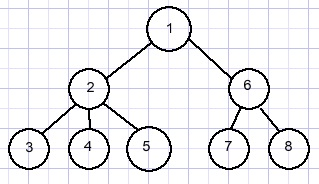Breadth first search always finds the shortest solution but in general
depth-first search finds a solution faster.
Reason is that breadth-first needs to take moves back a lot more times.

Move reduction

For each partial solution, the number of moves from start to end game positions is minimal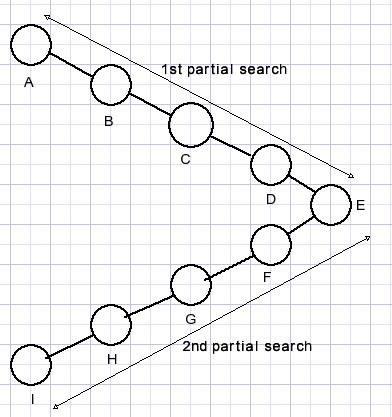The path from A to E is minimal and so is E to I.
However there may be a shorter path from A to F,G.. or C to F,G�.etc.
A shorter path from C to H eliminates board positions D,E,F,G while adding less other moves.
By varying the tile selection of partial solutions I found a 86 move solution
of the most difficult puzzle in a few minutes and a 84 move solution in an hour.
Addition: I now have a 78 move solution for the �extreme� puzzle.
Selection of higher search depths and selecting more tiles per partial solution will result in shorter solutions.

Studies mention that a 4*4 puzzle may be solved in 80 moves maximal,
however the examples show unsolvable puzzles.
Reason, I found out, is that in these solutions the empty space is located at the left top.

Xnr is the move number at search depth. Xnr is never decremented.
PXnr (Previous Xnr) differs from Xnr after taking back a move, so being at a previous board state.
Always PXnr <= Xnr.
A check for solution is only made if PXnr = Xnr
A timer is added for regular interruption of the search to communicate with the Windows operating system.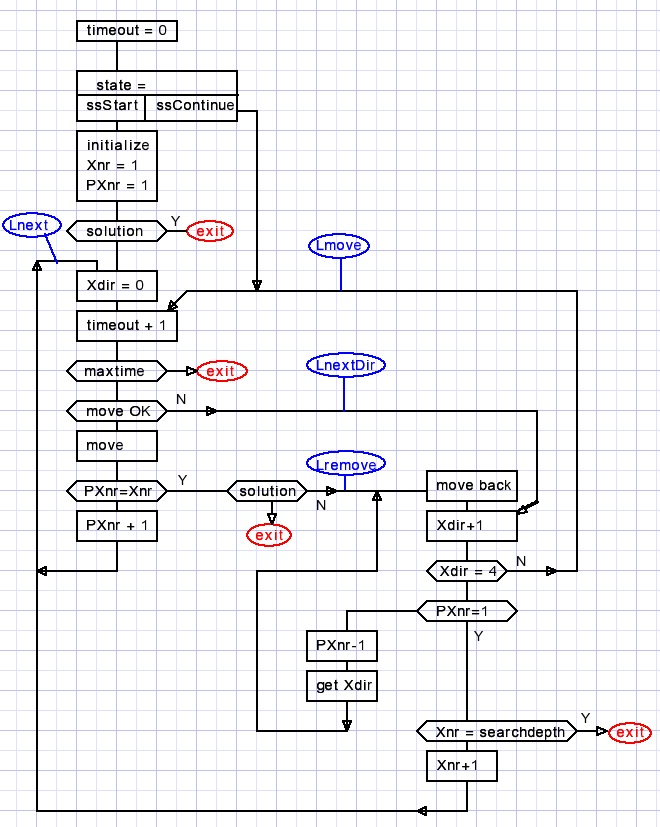Blue ovals are labels.

The search is initially started with ssStart followed by initialization of Xnr,PXnr,Xgame,Xdir.
After a time-out re-entry is with ssContinue.

This is the code to detect a partial solution:
```function testPartialSolution : boolean;
//test XGame for solution
var i : byte;
begin
result := true;
i := 1;
while result and (i < 16) do
begin
inc(i);
end;
end;
```
After a partially solved game, the XMove[ ] list with direction codes must be converted
to game states and added to the Game[.. , ..] array.

This procedure does the job :
```function UpdateGame : boolean;
//called after (partial) solved game
//insert Xmoves into game[..,..] array
var i,j,zf1,zf2 : byte;
begin
if moveNr + XNr > maxmove then begin
result := false;
exit;
end;
zf1 := Game[moveNr,0];
zf2 := zf1;
for i := 1 to 16 do XGame[i] := Game[moveNr,i];
for i := 1 to Xnr do
begin
inc(moveNr);
for j:= 1 to 16 do Game[moveNr,j] := Game[moveNr-1,j];
case Xmove[i] of
0 : zf2 := zf1 + 1;
1 : zf2 := zf1 - 4;
2 : zf2 := zf1 - 1;
3 : zf2 := zf1 + 4;
end;
Game[moveNr,0] := zf2;
Game[moveNr,zf1] := Game[moveNr,zf2];
Game[moveNr,zf2] := 0;
zf1 := zf2;
end;
result := true;
end;
```
A full solution in most cases will be a sequence of partial solutions.
To find a shorter solution, the procedure RBFsearch tries to find a shorter path
between two Game[ ] entries.
If found, procedure GReduce inserts the new game states in array Games[ ].The RBFSearch procedure is very similar to the Breadth first search procedure.
The spacing is the value of the search depth rotation button.
Please refer to the source code for details.

Program example: player move

The tile positions are stored in array game[0,0..16]
moveNr = 0; movecount = 0;
A tile is moved by a mouseclick on that tile.
A mousedown event on GImage calls function XYtoField with mouse (x,y) coordinates
and the field number 1..16 is returned.
Then procedure playermove is called with this field number.
If the maximal move was reached, the procedure playermove exits, nothing is done.

```procedure playermove(f1 : byte);//f1 is field number of mousedown
//--------------------------------------
zf := Game[moveNr,0];//zero field loaded in zf
n := abs(zf-f1);
if (n <> 1) and (n <> 4) then exit;//no adjacent empty field
//
m1 := moveNr + 1;
for n := 1 to 16 do Game[m1,n] := Game[moveNr,n];//copy to next
setMoveInfo(moveNr+1,moveNr+1);//update moveNr , movecount
game[moveNr,zf] := game[moveNr,f1];//do move
game[moveNr,f1] := 0;
game[moveNr,0] := f1;
slowmove(moveNr-1,moveNr);//tile moves to next board state
s := 'move '+inttostr(movecount);
if testsolution then
begin
s := s + ' game solved';
end;
form1.msglabel.Caption := s;
//------------------------------------
```
Procedure slowmove (in paint-unit) calls
```procedure paintTile(m,pos : byte; dx,dy : shortInt);
```
m is the move number (= index to game[ ] array.)
pos is the tile position (1..16)
dx is an offset to the tile X position.
dy is an offset to the tile Y position.
The sliding move is obtained by repeatedly calling painttile( ) with incrementing or decrementing values of dx,dy.
The speed of moving is set by calling
```procedure delay(msec : dword);//milliseconds elay
//use dav7timer1 on form1
begin
msec := msec * 1000;
with form1.dav7Timer1 do
begin
start;
repeat
application.ProcessMessages;
stop;
until Elapsedtime >= msec;//microseconds returned
end;
end
```
This concludes the 15-puzzle description.